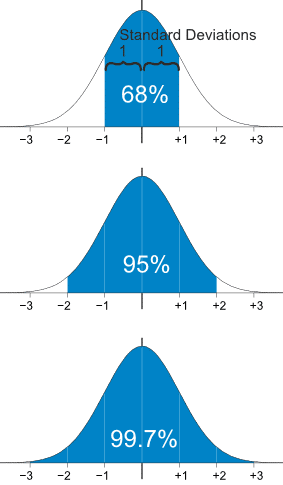# Why Standard Deviation Matters When Choosing Investments

Not all returns are created equal.

Standard deviation is a common statistical measurement and is defined by Oxford Dictionaries as:  ‘A quantity expressing by how much the members of a group differ from the mean value for the group.’ In other words, it is a measure of volatility that tells you how far apart all of the values are from the average (or mean) value.

For example, if Mutual Fund A has an average annual return of 10% and a standard deviation of 4%, you would expect about 68% of the time for the return to be between 6% and 14% (1 standard deviation on either side of 10%). About 95% of the time you would expect the return to be between 2% and 18% (or 2 standard deviations on either side of 10%).  This assumes the fund’s returns follow a normal distribution or what is commonly called a ‘bell curve.’ In a normal distribution, 68% of values are within 1 standard deviation of the mean, 95% of values are within 2 standard deviations of the mean and 99.7% of values are within 3 standard deviations of the mean.www.mathisfun.org

If Mutual Fund B has an average annual return of 10% and a standard deviation of 15%, you would expect about 68% of the time for the return to be between -5% and 25%.  About 95% of the time you would expect the return to be between -20% and 40%.

As you can see, not all returns are created equal and the volatility would be much greater with mutual fund B, even though they have the same average return. In my opinion, dealing with volatility often causes people to make decisions that may not be in their best interest. If you don’t think you would react well with a fund that has wild swings, perhaps you should consider one with a lower standard deviation.

Why Standard Deviation Matters When Choosing Investments appeared on http://rodgers-associates.com/blog/